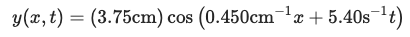# Problem: A water wave traveling in a straight line on a lake is described by the equationwhere y is the displacement perpendicular to the undisturbed surface of the lake.(a) How much time does it take for one complete wave pattern to go past a fisherman in a boat at anchor, and what horizontal distance does the wave crest travel in that time?(b) What are the wave number and the number of waves per second that pass the fisherman?(c) How fast does a wave crest travel past the fisherman, and what is the maximum speed of his cork floater as the wave causes it to bob up and down?

###### FREE Expert Solution

In a medium when the wave is traveling in the negative x-axis, the wave function y(x,t) that represents the displacement of the individual particles is given as:

$\overline{){\mathbf{y}}{\mathbf{\left(}}{\mathbf{x}}{\mathbf{,}}{\mathbf{t}}{\mathbf{\right)}}{\mathbf{=}}{\mathbf{A}}{\mathbf{c}}{\mathbf{o}}{\mathbf{s}}{\mathbf{\left(}}{\mathbf{k}}{\mathbf{x}}{\mathbf{+}}{\mathbf{\omega }}{\mathbf{t}}{\mathbf{\right)}}}$, where k is the wavenumber, A is the amplitude, x is the position of the string, ω is the angular frequency of the string, and t is the time taken.

The angular frequency of a wave:

$\overline{){\mathbf{\omega }}{\mathbf{=}}{\mathbf{2}}{\mathbf{\pi }}{\mathbf{f}}}$, where f is frequency.

(a)

From the equation of angular momentum and knowing that f =1/T, we can get the time period as:

$\overline{){\mathbf{T}}{\mathbf{=}}\frac{\mathbf{2}\mathbf{\pi }}{\mathbf{\omega }}}$

94% (293 ratings)###### Problem Details

A water wave traveling in a straight line on a lake is described by the equationwhere y is the displacement perpendicular to the undisturbed surface of the lake.
(a) How much time does it take for one complete wave pattern to go past a fisherman in a boat at anchor, and what horizontal distance does the wave crest travel in that time?
(b) What are the wave number and the number of waves per second that pass the fisherman?
(c) How fast does a wave crest travel past the fisherman, and what is the maximum speed of his cork floater as the wave causes it to bob up and down?

Frequently Asked Questions

What scientific concept do you need to know in order to solve this problem?

Our tutors have indicated that to solve this problem you will need to apply the Waves on a String concept. You can view video lessons to learn Waves on a String. Or if you need more Waves on a String practice, you can also practice Waves on a String practice problems.